# FlipFlops Latches Digital Electronics FlipFlops Latches This presentation

• Slides: 27Flip-Flops & Latches Digital Electronics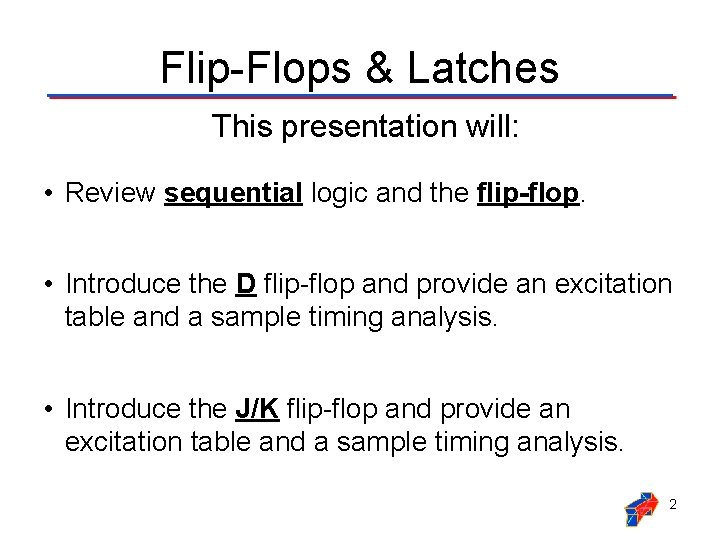Flip-Flops & Latches This presentation will: • Review sequential logic and the flip-flop. • Introduce the D flip-flop and provide an excitation table and a sample timing analysis. • Introduce the J/K flip-flop and provide an excitation table and a sample timing analysis. 2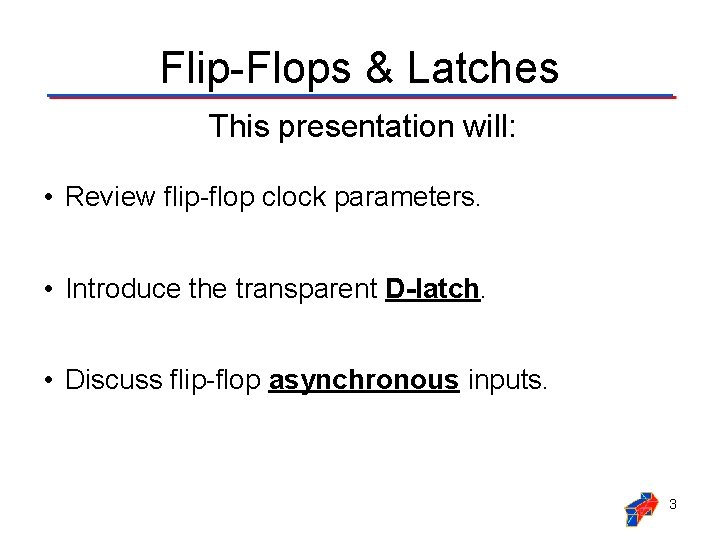Flip-Flops & Latches This presentation will: • Review flip-flop clock parameters. • Introduce the transparent D-latch. • Discuss flip-flop asynchronous inputs. 3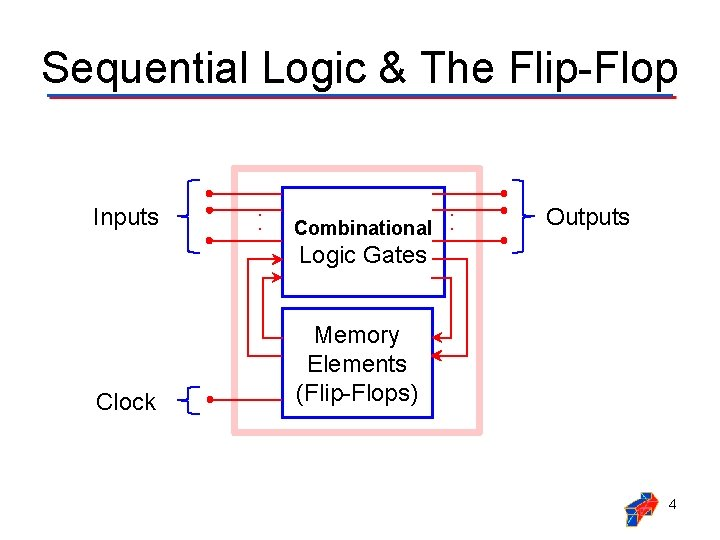Sequential Logic & The Flip-Flop Inputs . . Combinational . . Outputs Logic Gates Clock Memory Elements (Flip-Flops) 4D Flip-Flop: Excitation Table D CLK Q D CLK 0 0 1 1 1 0 : Rising Edge of Clock 5D Flip-Flop: Example Timing Q=D=0 Q=D=1 Q=D=0 No Change Q=D=0 Q=D=1 No Change Q=D=0 No Change Q D CLK 6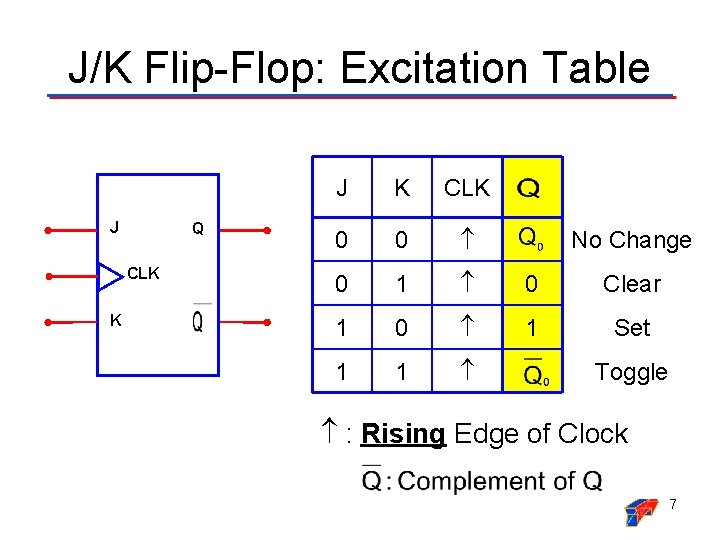J/K Flip-Flop: Excitation Table J Q CLK K J K CLK 0 0 0 1 0 Clear 1 0 1 Set 1 1 No Change Toggle : Rising Edge of Clock 7J/K Flip-Flop: Example Timing TOGGLE SET CLEAR TOGGLE NO CHANGE SET NO CHANGE Q J K CLK 8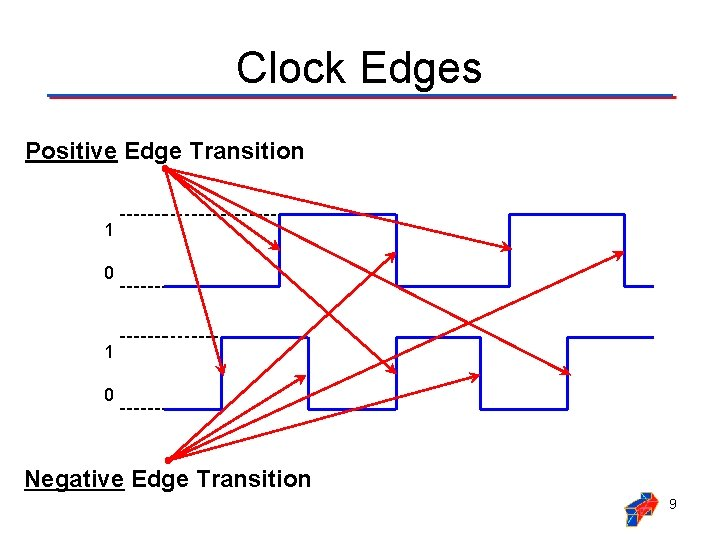Clock Edges Positive Edge Transition 1 0 Negative Edge Transition 9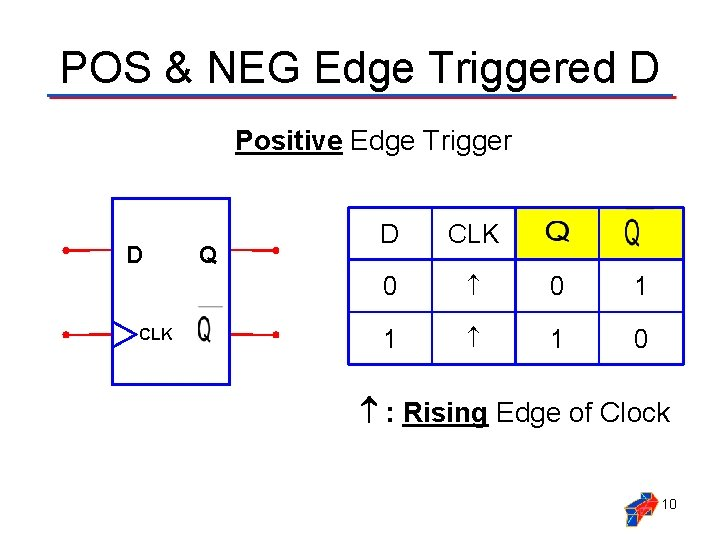POS & NEG Edge Triggered D Positive Edge Trigger D CLK Q D CLK 0 0 1 1 1 0 : Rising Edge of Clock 10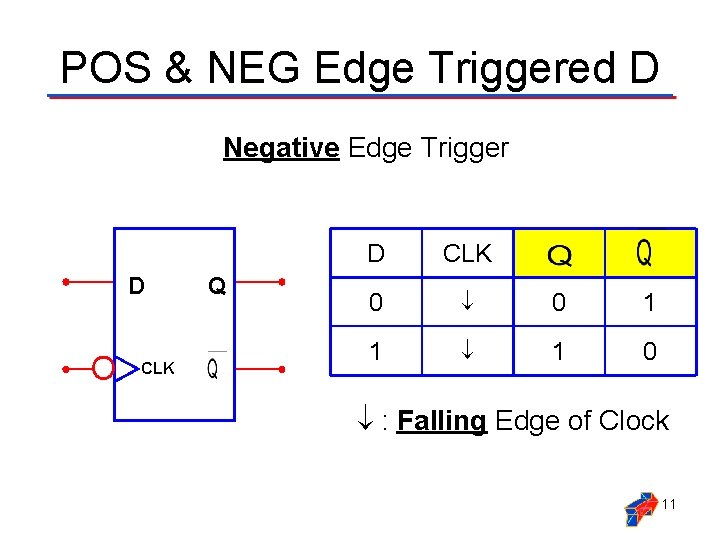POS & NEG Edge Triggered D Negative Edge Trigger D CLK Q D CLK 0 0 1 1 1 0 : Falling Edge of Clock 11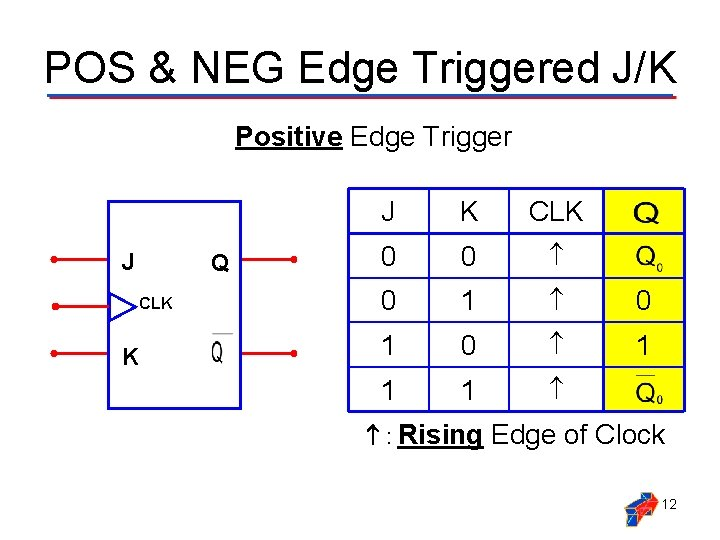POS & NEG Edge Triggered J/K Positive Edge Trigger J Q CLK K J K CLK 0 0 0 1 0 1 1 1 : Rising Edge of Clock 12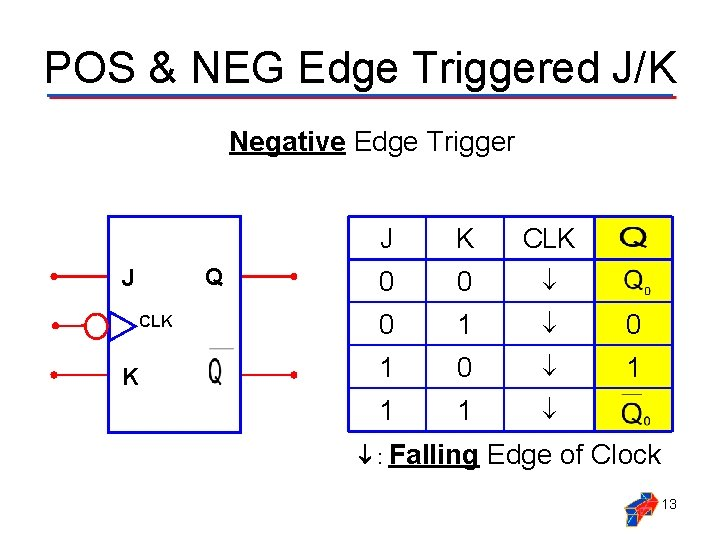POS & NEG Edge Triggered J/K Negative Edge Trigger Q J CLK K J K CLK 0 0 0 1 0 1 1 1 : Falling Edge of Clock 13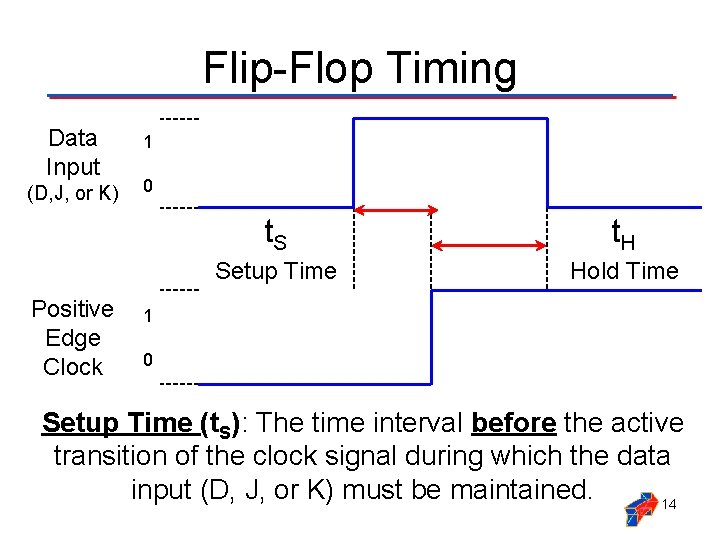Flip-Flop Timing Data Input (D, J, or K) Positive Edge Clock 1 0 t. S t. H Setup Time Hold Time 1 0 Setup Time (t. S): The time interval before the active transition of the clock signal during which the data input (D, J, or K) must be maintained. 14Flip-Flop Timing Data Input (D, J, or K) Positive Edge Clock 1 0 t. S t. H Setup Time Hold Time 1 0 Hold Time (t. H): The time interval after the active transition of the clock signal during which the data input (D, J, or K) must be maintained. 15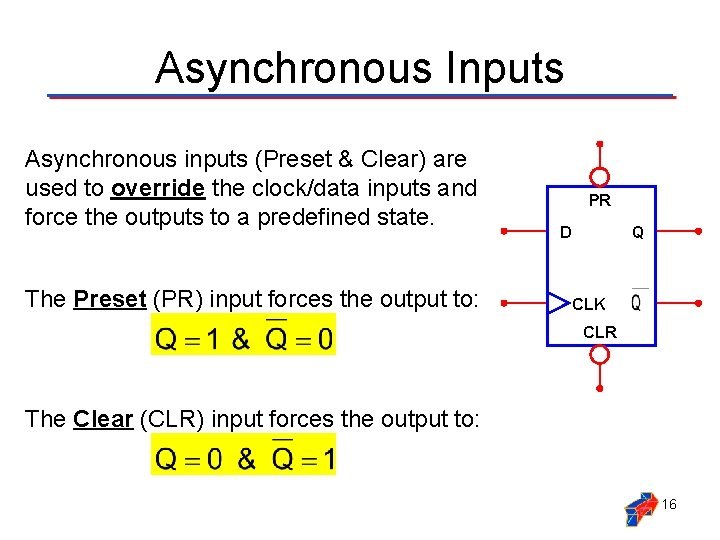Asynchronous Inputs Asynchronous inputs (Preset & Clear) are used to override the clock/data inputs and force the outputs to a predefined state. The Preset (PR) input forces the output to: PR D Q CLK CLR The Clear (CLR) input forces the output to: 16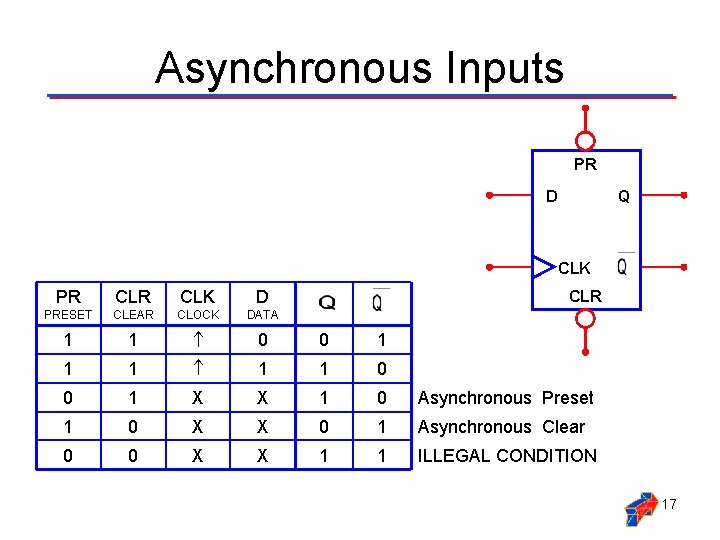Asynchronous Inputs PR D Q CLK PR CLK D PRESET CLEAR CLOCK DATA 1 1 0 0 1 X X 1 0 Asynchronous Preset 1 0 X X 0 1 Asynchronous Clear 0 0 X X 1 1 ILLEGAL CONDITION CLR 17D Flip-Flop: PR & CLR Timing Q=D=1 Q=D=0 Clocked Q PR Q=D=0 Q=D=1 Q=D=0 Clocked Q=1 Preset Q=0 Clear CLR D CLK 18Transparent D-Latch D EN Q EN D 0 X 1 0 0 1 1 0 EN: Enable 19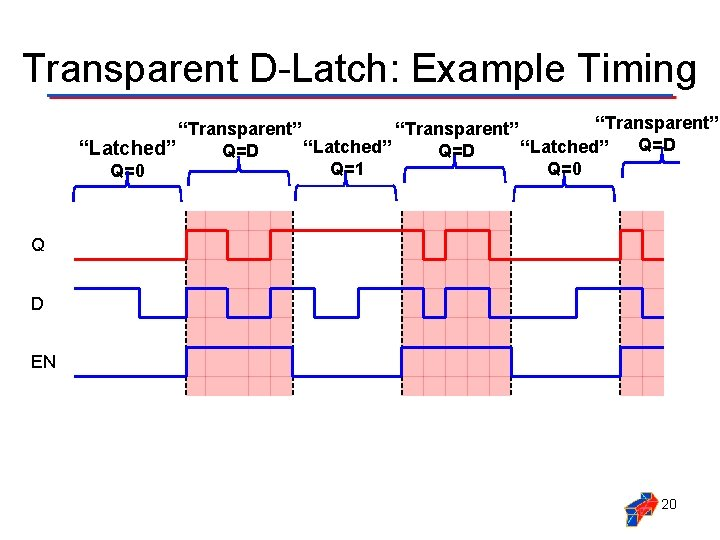Transparent D-Latch: Example Timing “Transparent” Q=D “Latched” Q=D Q=1 Q=0 Q D EN 20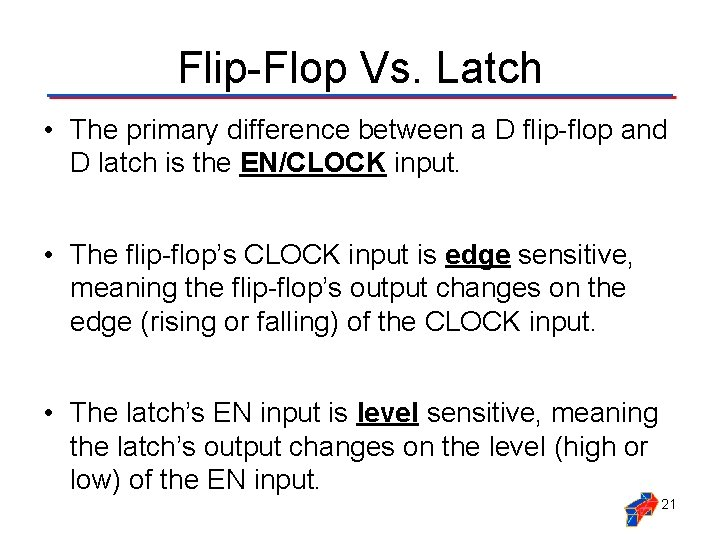Flip-Flop Vs. Latch • The primary difference between a D flip-flop and D latch is the EN/CLOCK input. • The flip-flop’s CLOCK input is edge sensitive, meaning the flip-flop’s output changes on the edge (rising or falling) of the CLOCK input. • The latch’s EN input is level sensitive, meaning the latch’s output changes on the level (high or low) of the EN input. 21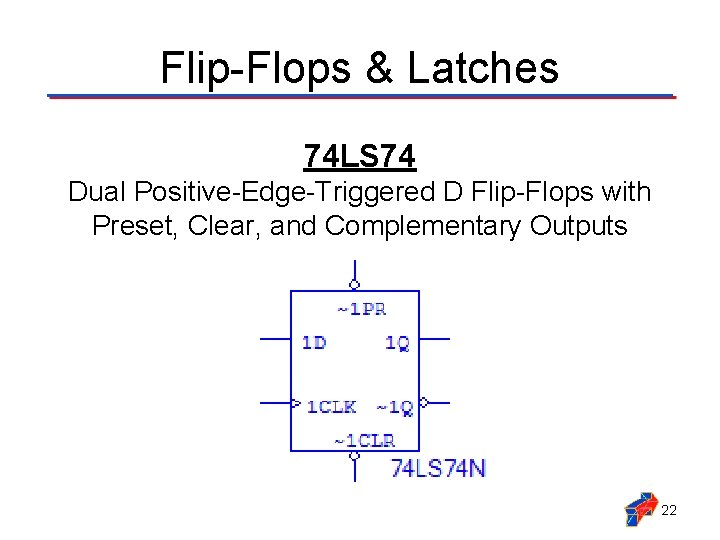Flip-Flops & Latches 74 LS 74 Dual Positive-Edge-Triggered D Flip-Flops with Preset, Clear, and Complementary Outputs 22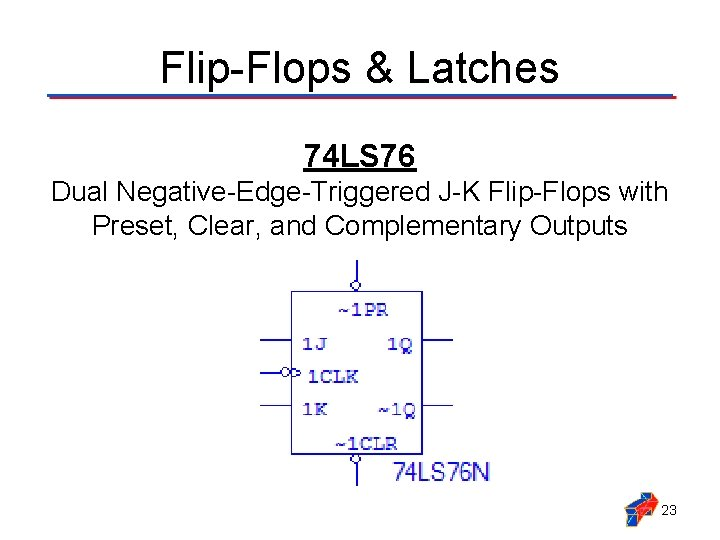Flip-Flops & Latches 74 LS 76 Dual Negative-Edge-Triggered J-K Flip-Flops with Preset, Clear, and Complementary Outputs 23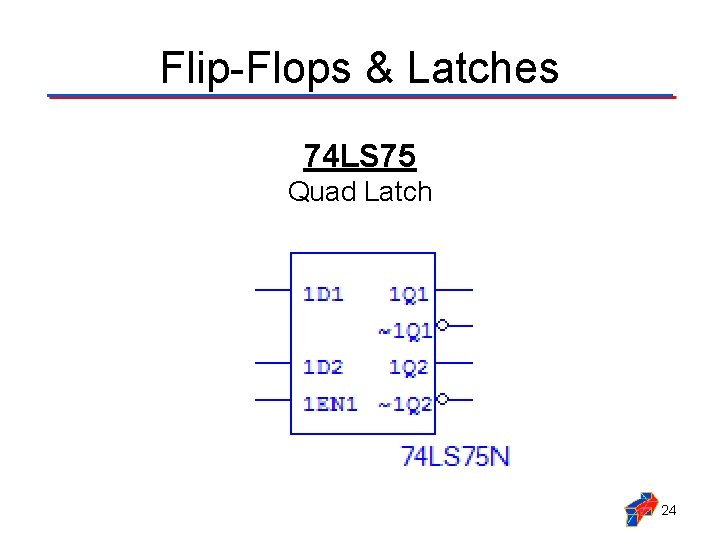Flip-Flops & Latches 74 LS 75 Quad Latch 2474 LS 74: D Flip-Flop 2574 LS 76: J/K Flip-Flop 2674 LS 75: D Latch 27